Gauss Law And Field Due To Charge Distribution MCQ

# Gauss Law And Field Due To Charge Distribution MCQ

Test Description

## 10 Questions MCQ Test Topic wise Tests for IIT JAM Physics | Gauss Law And Field Due To Charge Distribution MCQ

Gauss Law And Field Due To Charge Distribution MCQ for IIT JAM 2023 is part of Topic wise Tests for IIT JAM Physics preparation. The Gauss Law And Field Due To Charge Distribution MCQ questions and answers have been prepared according to the IIT JAM exam syllabus.The Gauss Law And Field Due To Charge Distribution MCQ MCQs are made for IIT JAM 2023 Exam. Find important definitions, questions, notes, meanings, examples, exercises, MCQs and online tests for Gauss Law And Field Due To Charge Distribution MCQ below.
Solutions of Gauss Law And Field Due To Charge Distribution MCQ questions in English are available as part of our Topic wise Tests for IIT JAM Physics for IIT JAM & Gauss Law And Field Due To Charge Distribution MCQ solutions in Hindi for Topic wise Tests for IIT JAM Physics course. Download more important topics, notes, lectures and mock test series for IIT JAM Exam by signing up for free. Attempt Gauss Law And Field Due To Charge Distribution MCQ | 10 questions in 30 minutes | Mock test for IIT JAM preparation | Free important questions MCQ to study Topic wise Tests for IIT JAM Physics for IIT JAM Exam | Download free PDF with solutions
 1 Crore+ students have signed up on EduRev. Have you?
Gauss Law And Field Due To Charge Distribution MCQ - Question 1

### If the flux of the electric field through a closed surface is zero

Detailed Solution for Gauss Law And Field Due To Charge Distribution MCQ - Question 1

Flux across a closed surface,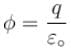Since,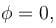so, charge enclosed q = 0

The correct answer is: the net charge inside the surface must be zero

Gauss Law And Field Due To Charge Distribution MCQ - Question 2

### Electric charge is uniformly distributed along a long straight wire of radius 1mm. The charge per cm length of the wire is Q  coulomb. Another cylindrical surface of radius 50cm and length 1m symmetrically encloses the wire as shown in figure. The total electric flux passing through the cylinder surface is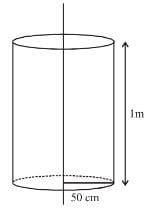Detailed Solution for Gauss Law And Field Due To Charge Distribution MCQ - Question 2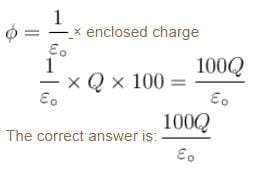Gauss Law And Field Due To Charge Distribution MCQ - Question 3

### A sphere of radius R has a uniform of volume charge density. The electric potential at a point r, r < R  is

Detailed Solution for Gauss Law And Field Due To Charge Distribution MCQ - Question 3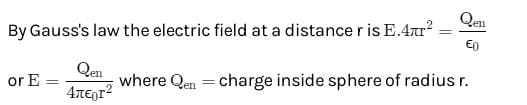As the potential V=−∫Edr so the electric potential at r due to charge inside a sphere of radius r only.

Gauss Law And Field Due To Charge Distribution MCQ - Question 4

Charge on the outer sphere is q and the inner sphere is grounded. Then the charge q' on the inner sphere r2 > r1.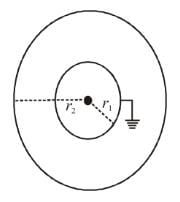Detailed Solution for Gauss Law And Field Due To Charge Distribution MCQ - Question 4

Potential at the surface of inner sphere = 0 (grounded)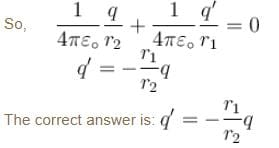Gauss Law And Field Due To Charge Distribution MCQ - Question 5

If the electric flux entering and leaving an enclosed surface respectively is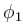and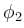the electric charge inside the surface will be

Detailed Solution for Gauss Law And Field Due To Charge Distribution MCQ - Question 5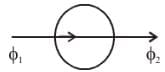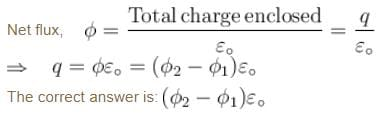Gauss Law And Field Due To Charge Distribution MCQ - Question 6

The SI unit of electric flux is

Detailed Solution for Gauss Law And Field Due To Charge Distribution MCQ - Question 6

Electric flux  = E.A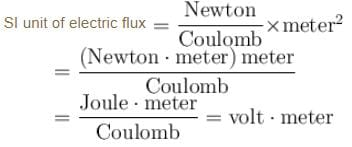The correct answer is: volt × metre

Gauss Law And Field Due To Charge Distribution MCQ - Question 7

A point charge is kept at the mid point between two large parallel grounded conducting plates. Assuming no gravity. The charge is displaced a little towards the right plate. The charge will now

Detailed Solution for Gauss Law And Field Due To Charge Distribution MCQ - Question 7

When the charge is placed at the mid point, opposite charge will induced on both the plates equally. But when q is displaced toward the right, more charge of the opposite sign will get induced on the right plate than on the left plate. Hence the point charge will feel a net attraction towards the right plate.

The correct answer is: Move towards the right plate

Gauss Law And Field Due To Charge Distribution MCQ - Question 8

The figure shows electric field E at a distance r in any direction from the origin O. The electric field is due to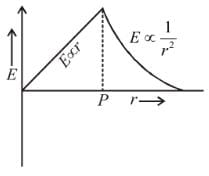Detailed Solution for Gauss Law And Field Due To Charge Distribution MCQ - Question 8

From Gauss Law of electrostatics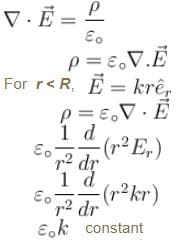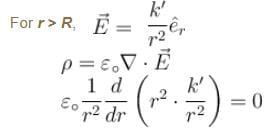The correct answer is: a uniform charged non-conducting sphere of radius OP  with centre at O.

Gauss Law And Field Due To Charge Distribution MCQ - Question 9

A charge Q is enclosed by a Gaussian spherical surface of radius R. If the radius is doubled, then the outward electric flux will

Detailed Solution for Gauss Law And Field Due To Charge Distribution MCQ - Question 9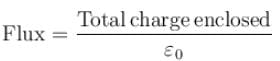Since, flux across a closed surface depends only on the charge enclosed. So, it will remain same

The correct answer is: remain the same

Gauss Law And Field Due To Charge Distribution MCQ - Question 10

Given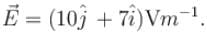The electric flux through 1m2 area in YZ plane is

Detailed Solution for Gauss Law And Field Due To Charge Distribution MCQ - Question 10

For yz plane,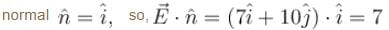So, flux across yz plane is given by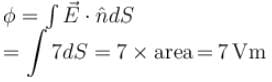## Topic wise Tests for IIT JAM Physics

217 tests
 Use Code STAYHOME200 and get INR 200 additional OFF Use Coupon Code
Information about Gauss Law And Field Due To Charge Distribution MCQ Page
In this test you can find the Exam questions for Gauss Law And Field Due To Charge Distribution MCQ solved & explained in the simplest way possible. Besides giving Questions and answers for Gauss Law And Field Due To Charge Distribution MCQ, EduRev gives you an ample number of Online tests for practice

217 tests